# Explained: Coast Guard Geographic Range Table promoted by flat earthers

Discussion in 'Flat Earth' started by Bunkmeister, Aug 22, 2017.

1. ### BunkmeisterNew Member

In discussing horizons and visibility with flat earthers, a US Coast Guard geographic range table was brought to my attention. This is a guide for estimating visibility distances over open water.

The full document that this came from here is here on page 7 (https://www.navcen.uscg.gov/pdf/lightLists/LightList V6.pdf) but I have attached the relevant portion below.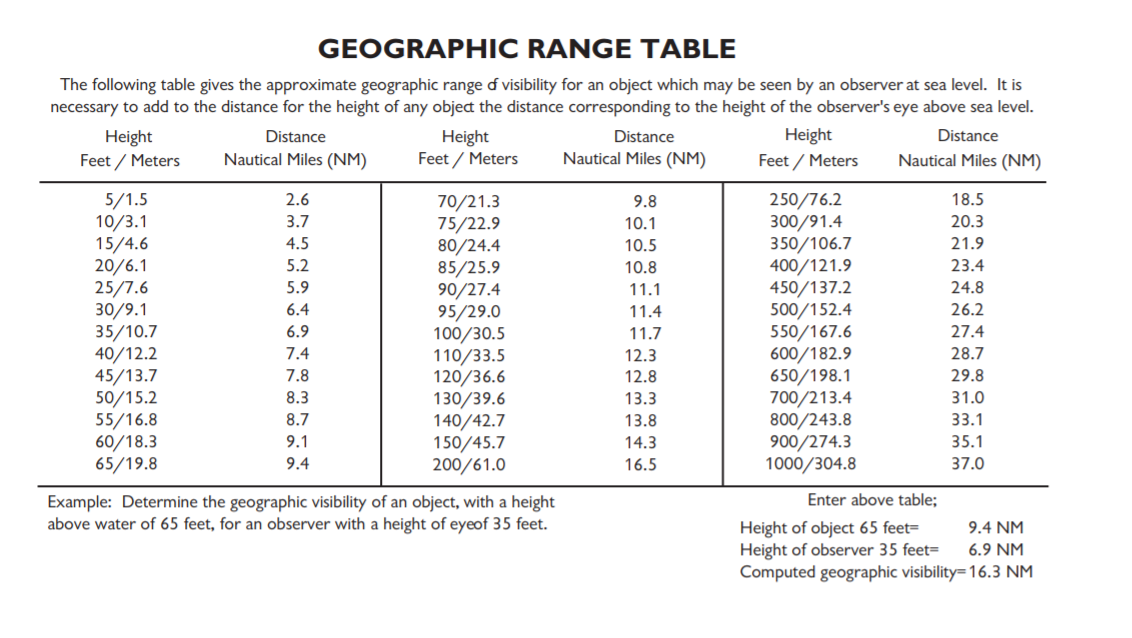As I read this, one takes the provided nautical miles distance of the observer height and the object height, adds them together, and can get a quick estimate of visibility range.

In the sample example provided above, the observer height is 35 feet, object height is 65 feet, and the computed geographic visibility is 16.3 nautical miles (18.76 regular miles). I plugged in 18.76 miles to the curve calculator, and 88 feet is the amount hidden, a full 23 feet more than the purported object. Does this not contradict generally accepted notions of curvature? Am I missing something?

2. ### Mick WestAdministratorStaff Member

The table shows the practical distance to the horizon including average refraction. The extra value used for average refraction varies a little (as it varies a lot in real life, depending on the weather), but is around 1/7 of the geometric distance. So from 65 feet it's 9.5 NM, and from 35 feet it's 6.5 NM. 1 NM is 1.15 miles, so that's 10.9 miles and 7.5 miles.

With my curve calculator it's 9.9 miles, 10.7 with refraction (vs. 10.9)
https://www.metabunk.org/curve/?d=0&h=65&r=3959&u=i&a=n&fd=60&fp=3264

and then 7.2 miles and 7.8 with refraction (vs. 7.5)
https://www.metabunk.org/curve/?d=0&h=35&r=3959&u=i&a=n&fd=60&fp=3264

If you plug in the total distance of 18.76 miles from 35 feed you get:
https://www.metabunk.org/curve/?d=18.76&h=35&r=3959&u=i&a=n&fd=60&fp=3264
Distance = 18.76 Miles (99052.8 Feet), View Height = 35 Feet (420 Inches) Radius = 3959 Miles (20903520 Feet)

Results ignoring refraction
Horizon = 7.24 Miles (38252.42 Feet)
Bulge = 58.67 Feet (704.05 Inches)
Drop = 234.69 Feet (2816.23 Inches)
Hidden= 88.42 Feet (1061.07 Inches)
Horizon Dip = 0.105 Degrees, (0.0018 Radians)

With Standard Refraction 7/6*r, radius = 4618.83 Miles (24387440 Feet)
Refracted Horizon = 7.83 Miles (41317.33 Feet)
Refracted Drop= 201.16 Feet (2413.91 Inches)
Refracted Hidden= 68.34 Feet (820.11 Inches)
Refracted Dip = 0.097 Degrees, (0.0017 Radians)

The refracted hidden is 68 feet (vs. 65 feet). So that seems about right, as the visibility table would include refraction as it's intend to be what you can actually see, not some theoretical geometric visibility.

3. ### Mick WestAdministratorStaff Member

Perhaps the more relevant issue here is: why does the table describes visibility over a curved surface? What exactly is the point of a Flat Earther bringing up this table if all it says is that the effective radius of the globe (if we ignore refraction) is slightly bigger than expected. Are they just "slightly bigger globe-heads"?

How was this table used in conversation?

•Funny x 2
4. ### BunkmeisterNew Member

It was posted during a debate about whether curved water exists. I was told that this was bombshell information from the government itself contradicting the globe earthers' claims about curvature of the earth.

I just noticed this piece within the last week. Not sure if this is a new meme, but it would not surprise me if you start to see this starting to pop up from flat-earthers more often. It has been posted multiple times on one of the largest flat earth Facebook groups.

5. ### Z.W. WolfSenior Member

Could you give us a few first hand examples?

These tables have been used for hundreds of years, btw.

Brief history of the American Practical Navigator [or Bowditch] from Wiki:

These distance of visibility tables aren't the only thing in Bowditch, and not all distance of visibility tables are from Bowditch; I'm just giving you an idea of how long they have been in use. These charts have been used millions of times in association with navigation techniques that only work on a spherical surface. So bringing up this whole subject would seem to be pretty self-defeating for any FE believer. But maybe they're just trying to point out an anomaly. Any anomaly.

In this 1943 US Navy training film - Night Piloting -student navigators are taught to refer to:Table 8 - Distance of Visibility of Objects at Sea: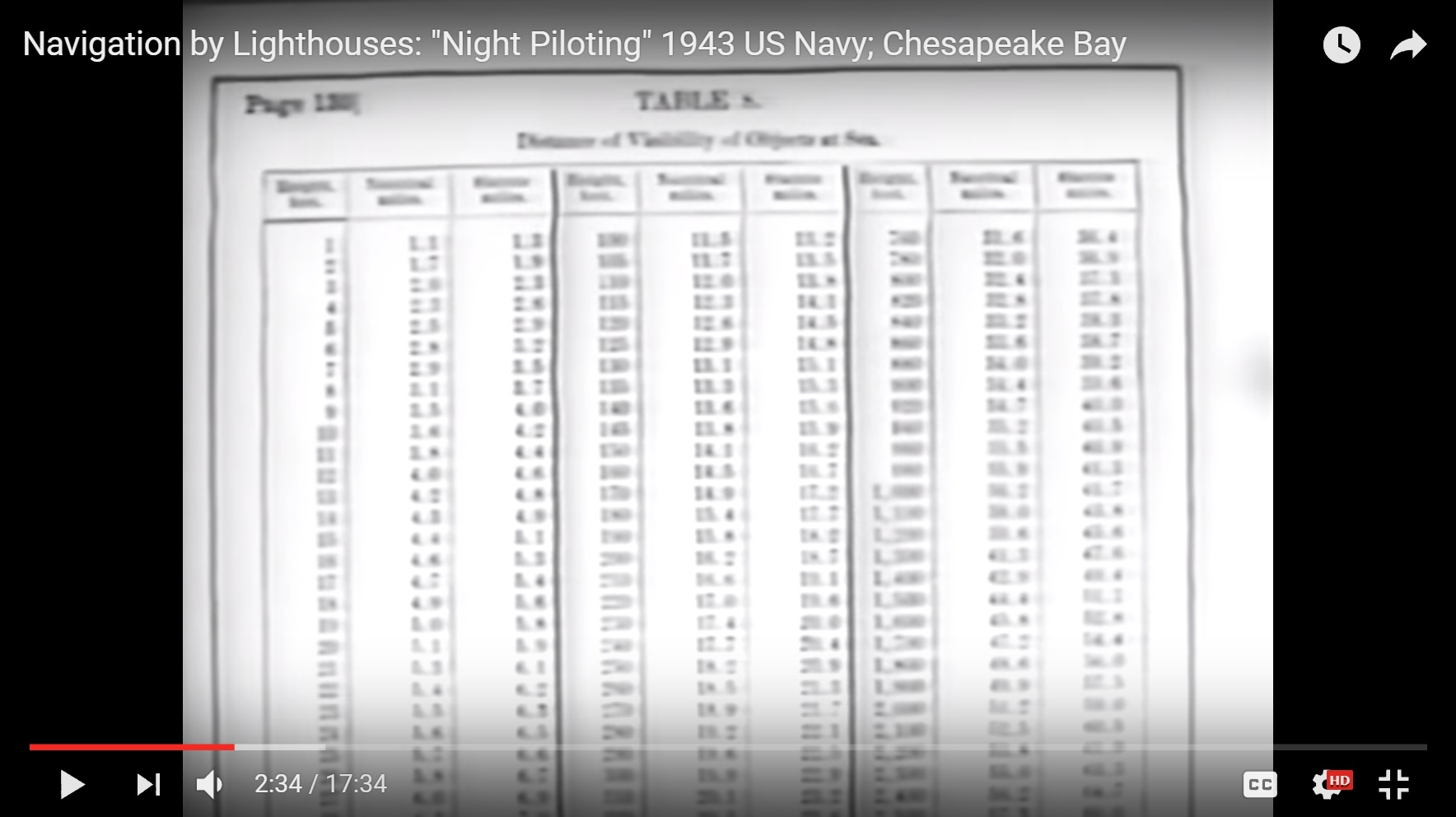Last edited: Aug 22, 2017
6. ### Z.W. WolfSenior Member

Bobbing the light. One of our members describes practical experience at sea:

https://www.metabunk.org/earth-curv...bunking-flat-concave-earth.t6042/#post-149495

An explanation of the technique from: http://boatsafe.com/nauticalknowhow/distance.htm

You can use tables or you can use a formula:

Last edited: Aug 22, 2017
•Like x 2

Examples of?

8. ### Z.W. WolfSenior Member

... of the arguments being presented by FE believers. You've given us a second hand account. Can you give us a first hand example? I just want to be sure what it is they are actually arguing.

Is this just one isolated thing you've run across, or are there more?

Last edited: Aug 22, 2017
9. ### RorySenior Member

And do they explain why being higher helps them see further?

10. ### VoysovReasonNew Member

Here is a first hand example of a Flat Earther using this chart.

Nathan Oakley promotes this chart as proof of flat earth at the FEIC conference in Denver, in 2018.

Here is the video, go to about 2:44:00

Source: https://youtu.be/oz35aaxJTik

11. ### Mick WestAdministratorStaff Member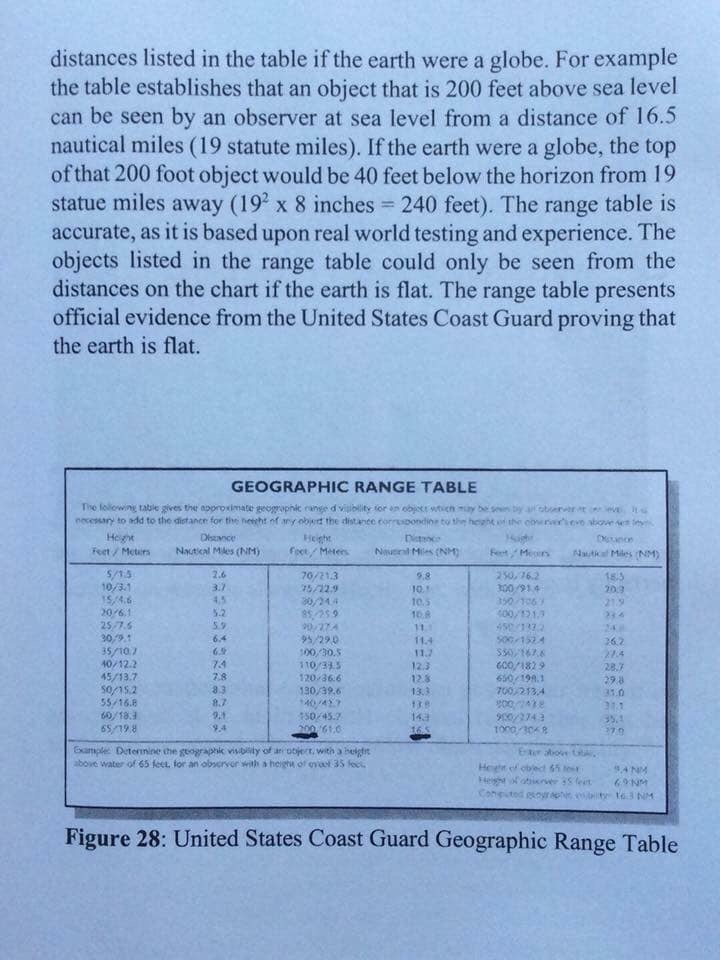Example of it being used, presumable in some FE book.

12. ### M. AndersonNew Member

The table and equation are based on a spherical earth, especially when you look at the derivation of the equation.

The horizon distance (H) on a globe with radius (R) and observer height (X) can be accurately calculated with the right triangle rule. However this isn't easy to work out in your head and it requires both the observer height and radius to use the same units.

H = [(R+X)^2 - R^2]^1/2

If we factor out the exponent​
= [R^2 + 2*R*X + X^2 - R^2] ^1/2

The R^2 and -R^2 cancel each other out​
= [2*R*X + X^2]^1/2

Pull the X out​
= [(2*R + X) * X]^1/2

Which can be rewritten as​
= (2*R + X)^1/2 * X^1/2

The format of this looks pretty similar to the equation posted by Z.W. Wolf. In the expression (2*R + X), we know that R will be much much bigger than X, therefore lets assume X is 0 for this section. We then get...

H = (2*R + 0)^1/2 * X^1/2
= (2*R)^1/2 * X^1/2

Remember, this equation requires both X and R to have the same units, but the equation used by the table has an input of ft and output of Nmi. Lets say both R and X are in feet which will give the horizon distance in feet, so lets add a conversion to change the horizon distance to Nmi.

H = ((2*R)^1/2 * X^1/2) ft
= ((2*R)^1/2 * X^1/2) ft * (1Nmi / 6076ft)
= ((2*R)^1/2) / 6076 * X^1/2 Nmi

Since R is a constant, the first half of the equation will not change based on observer height. Now we have an equation that allows us to input the observer height X in ft, and we'll get out the Horizon distance in Nmi and we can do the calculation pretty easily in our head. If we use the 1.17 from the equation posted by Z.W. Wolf, we can calculate the value of R (in ft) used.

1.17 = ((2*R)^1/2) / 6076
R = [(1.17 * 6076)^ 2] / 2
R = 25268372 ft

Convert this to miles.

R = 4785.7 miles

The coast guard table is based on a sphere with radius 4786 miles. The standard refraction radius 7/6*R is 4618 miles. So the coast guard either assumes light bends even more than the standard refraction model (approx 1.2 instead of 1.16) or that the earth is bigger than conventionally thought. Either way, it does nothing to prove that the earth is flat.

13. ### Mick WestAdministratorStaff Member

Hah, I wrote this last week but did not hit “post”

Looking into this a little more, given that it's the distance to the horizon, you can use that to calculate the value of R they use. Not perfect as they have only one decimal place. But it turns out if you do a table of distance to horizon for R=1.21*3959 then the results are exactly the same.

Last edited: May 29, 2019

15. ### Mick WestAdministratorStaff Member

Still in use (Jennifer Brown is Nathan Thompson)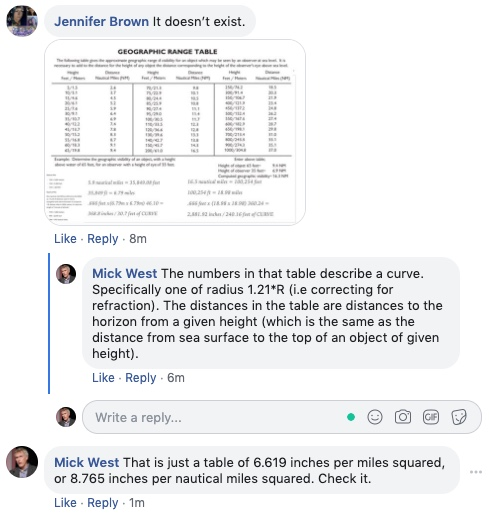16. ### Mick WestAdministratorStaff Member

Nathan also brought up the table as a globe-busting proof in our debate in Las Vegas (May 26, 2019). He genuinely did not seem to understand what I was saying, as evidenced by him continuing to use it.

Here's a plot of the values. The horizontal axis is Nautical Miles, vertical is height, which is essentially the same as "drop".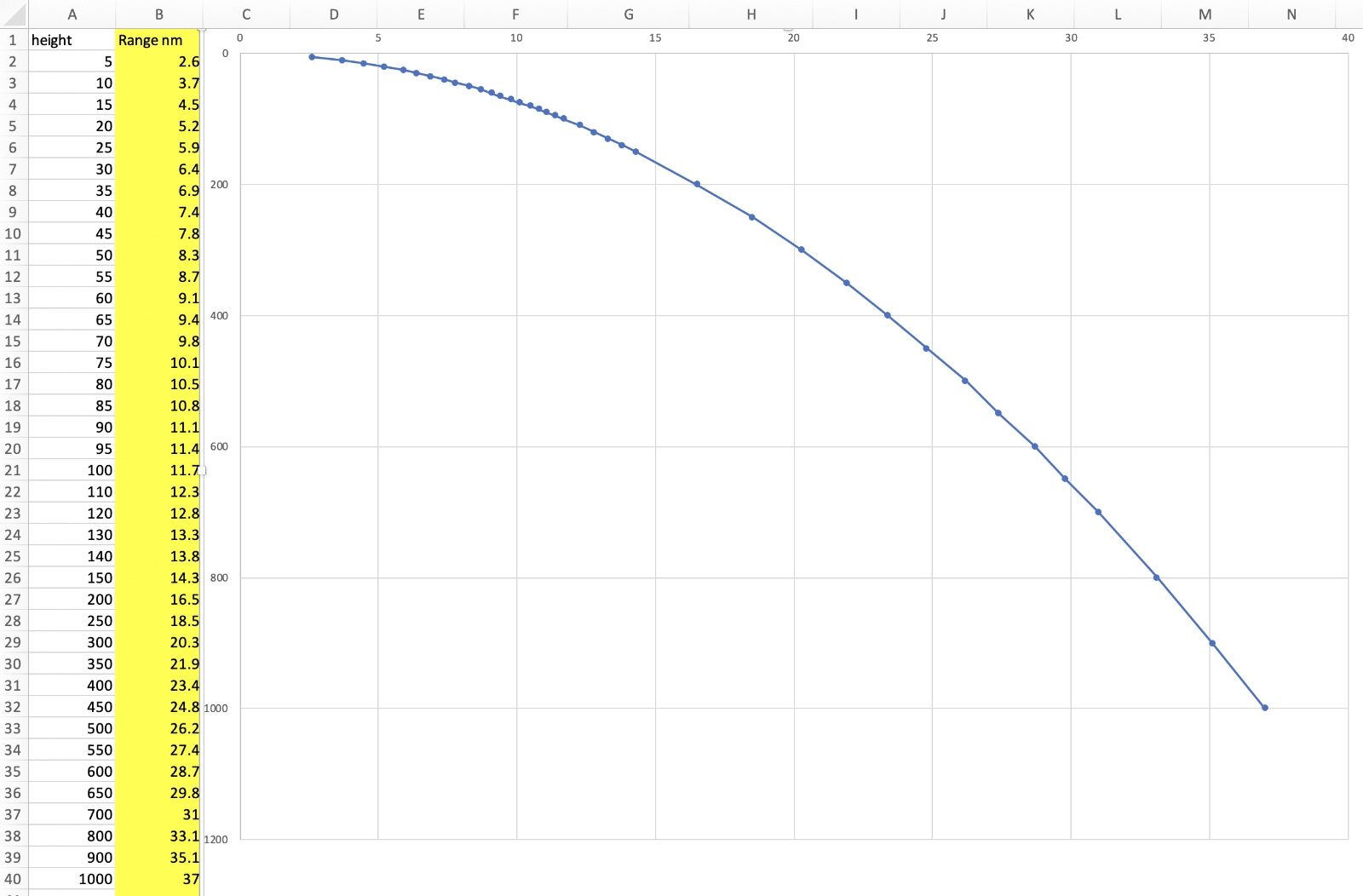File size:
19.4 KB
Views:
22